My last article covered spread syntax and `Object.assign` in detail, but glossed over rest parametersin the interest of time. I do, however, feel they deserve a closer look.

Let’s begin at the trusty MDN Docs:

The rest parameter syntax allows us to represent an indefinite number of arguments as an array.

That last part, “as an array”, is interesting, because before ES6 arrow functions, we used the `arguments` object. It was array-like, but not actually an array.

Example:

``````function returnArgs() {
return arguments;
}
``````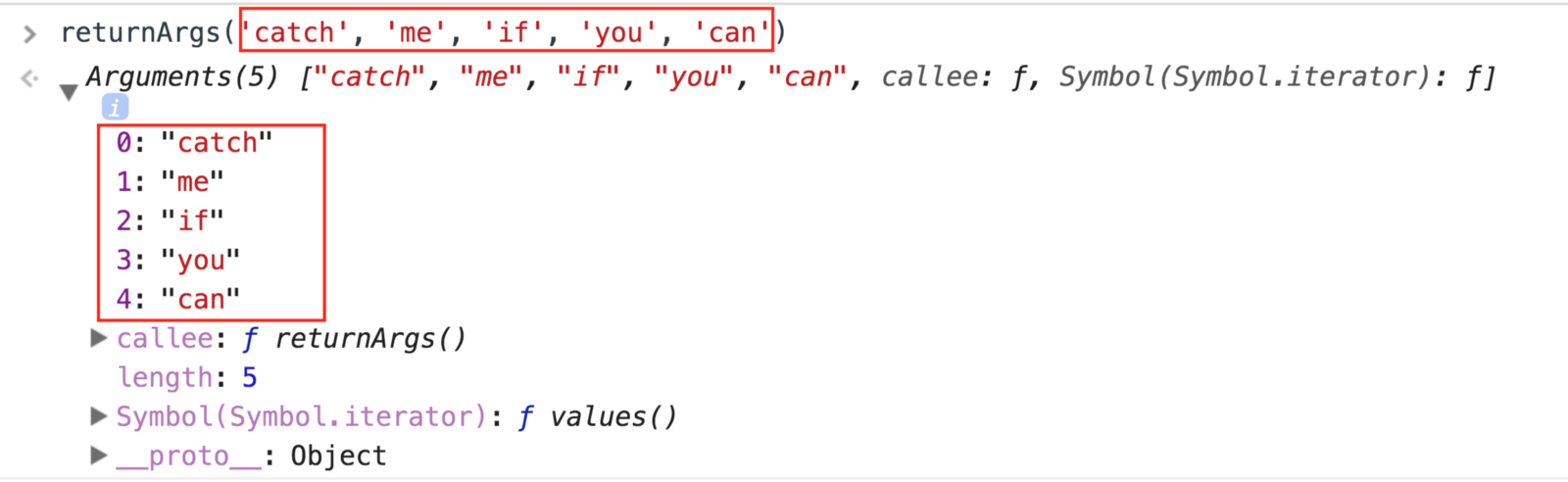We see `arguments` has indices, so it’s loop-able:

``````function loopThruArgs() {
let i = 0;

for (i; i < arguments.length; i++) {
console.log(arguments[i]);
}
}
``````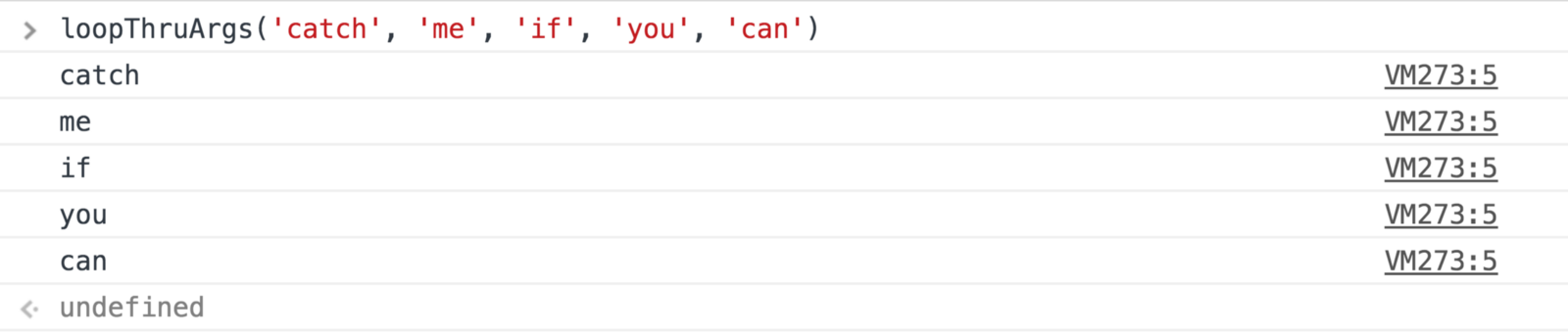But it’s not an array.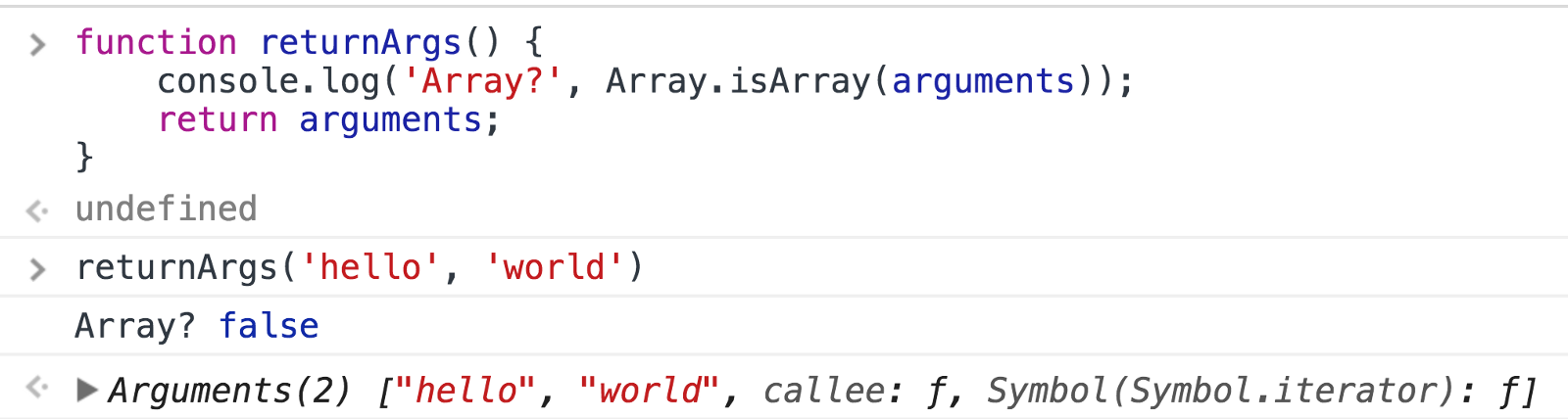Let’s contrast that with a function using rest parameters:

``````es6Params = (...params) => {
console.log('Array?', Array.isArray(params));
return params;
};
``````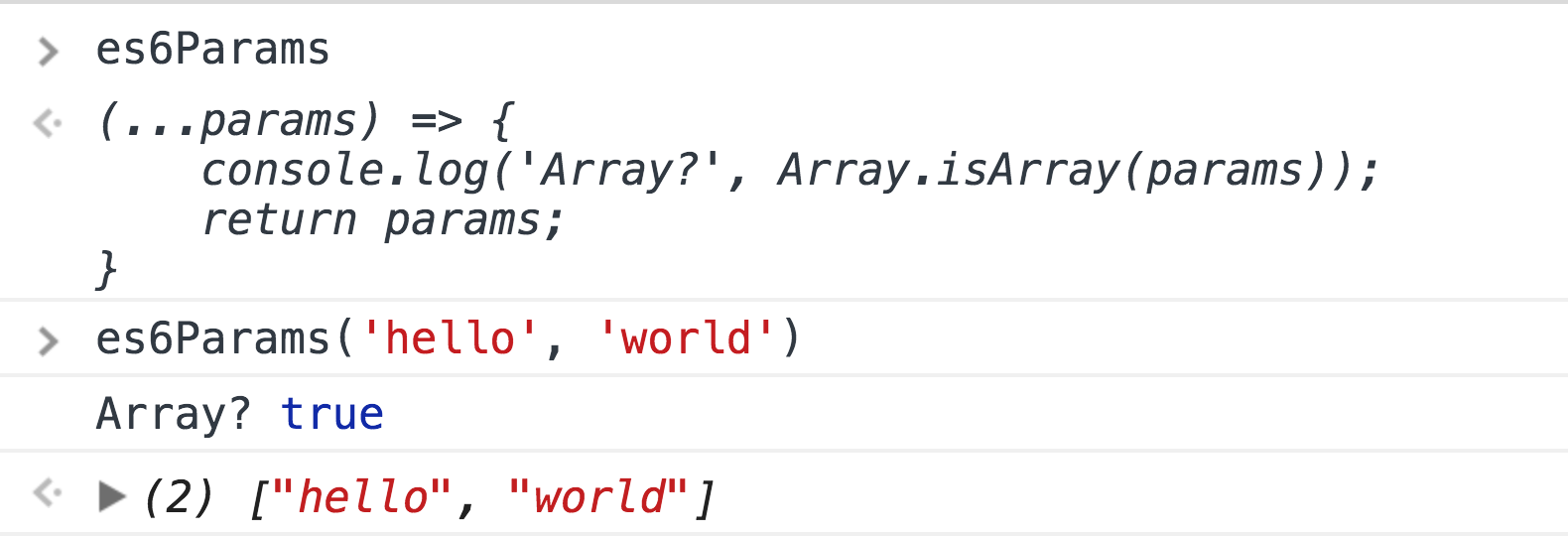It’s just an array, meaning we can use any of the `Array` methods on it!

Let’s write a function that doubles and sums every parameter you give it.

``````double = (x) => x * 2;
sum = (x, y) => x + y;

doubleAndSum = (...numbers) => numbers.map(double).reduce(sum, 0);
``````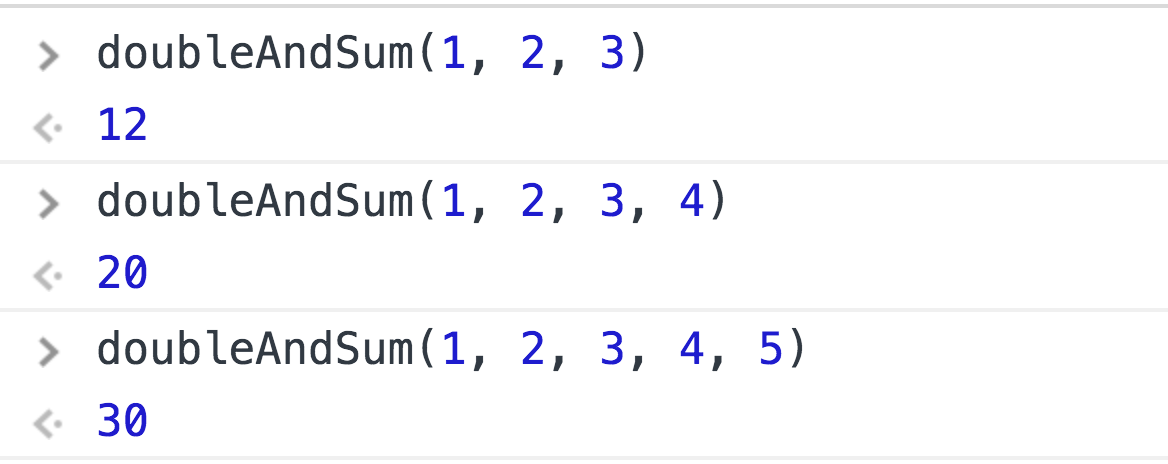And you can name as many parameters as you want in your function before using rest.

``````someFunction = (a, b, c, ...others) => {
console.log(a, b, c, others);
};
``````But it has to be the last one specified, since it captures the rest of your arguments. ?I think we know what’s happening under the hood, but let’s be thorough. Check out babeljs.io/repl, where you can write ES6+ code and have it transpiled into ES5 in real-time.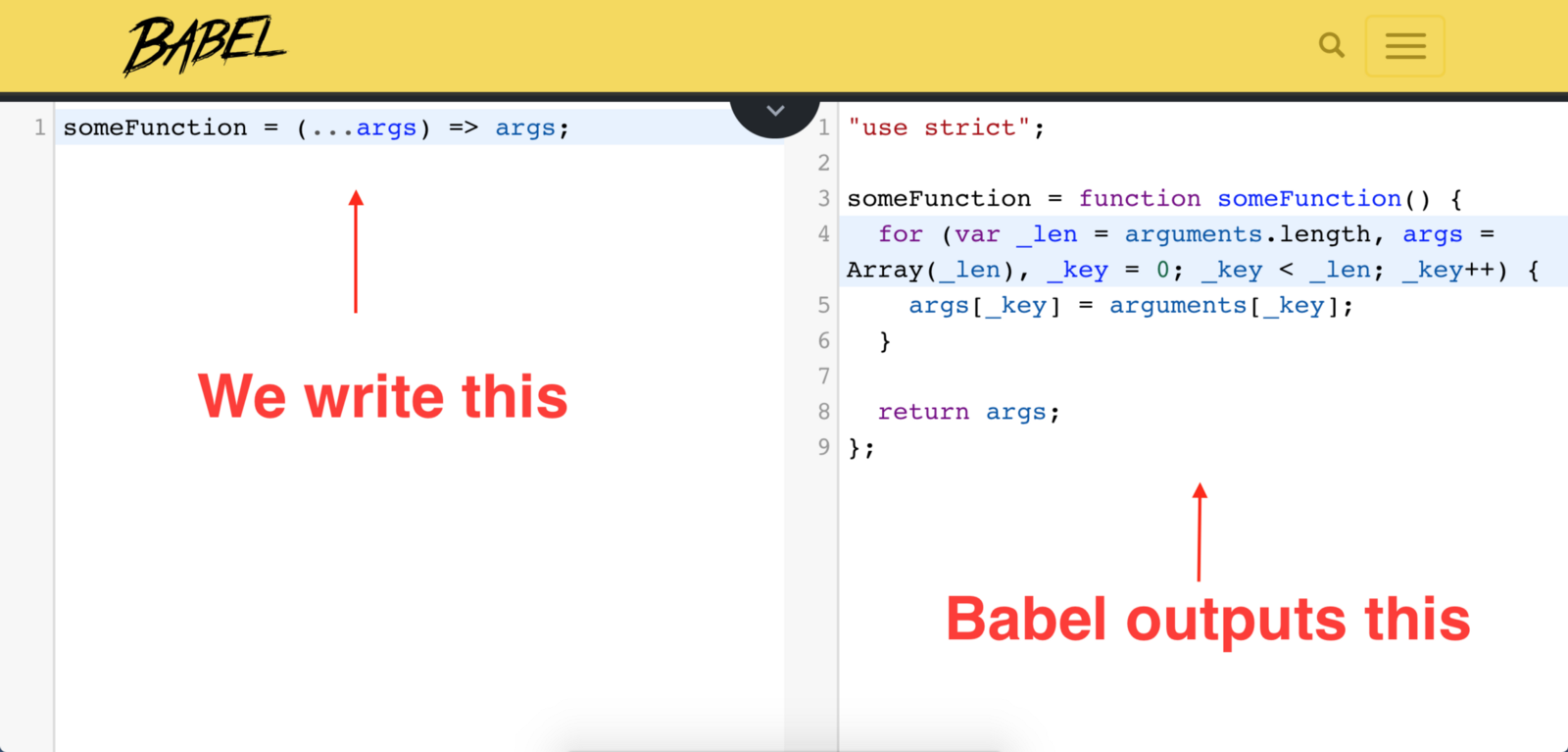``````someFunction = function someFunction() {
var _len = arguments.length;

// create an array same length
// as the arguments object
var args = Array(_len);
var i = 0;

// iterate through arguments
for (i; i < _len; i++) {
// assign them to
// the new array
args[i] = arguments[i];
}

// and return it
return args;
};
``````

Since Babel wrote an old-school function for us, it can access the `arguments` object! `arguments` has indices and a `.length` property, which is all we need to create a perfect clone of it.

This is why we can use Array methods like `map`, `filter`, `reduce` on rest parameters, because it creates an Array clone of `arguments`.

Have fun rest-ing!